Chris Pollett > Students >
Newth

( Print View )

[Bio]

[GJK]

GJK: Fast Convex Hull Collision Detection in 2D and 3D

As part of my new job and my thesis I researched and implemented a 2D version of GJK (Gilbert-Johnson-Keerthi), a useful and fast collision detection algorithm. I will attempt to explain its operation and provide a basic implementation that is consistent with the excellent presentation found at MollyRocket (link provided below) as as well as providing an explanation of what one does after detecting a collision using GJK.

The Minkowski Difference

The basic tool of GJK is the Minkowski Difference, explained in more depth and to far greater benefit by Wikipedia and this site. The Minkowski difference is an inherently graphical concept. To see it is to know it immediately; To read about it is a lot less useful. Here goes: Think of it as an object where each point is the result of a point in object A - a point in object B.

Minkowski (x, y) = (Ax - Bx, Ay - By)

For the purposes of finding collisions one can simply consider the vertices of each object, but it is important to remember that we are talking about the infinite number of points that make up the 2D area (or in 3D, the volume) of each object. It is also worth drawing out a few to get the concept fixed in your brain. Heres a screenshot of Paul Firth's applet: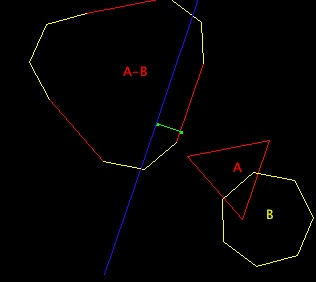The weird object is the Minkowski Difference of the triangle and heptagon. We also note that if two objects are overlapping then there exists at least one point on each object A and B are the same, so the equation becomes:

Minkowski point (x, y) = (Ax - Bx, Ay - By) = (0, 0)

This means that if two convex hulls collide, then the Minkowski Difference of the two objects contains the origin. GJK is how to implement the question: Can I draw a simplex using only points on the surface of the Minkowski Difference object that contain the origin? If so, I have detected a collision. If not, then there is no collision. In 2D, a simplex means a triangle and in 3D simplex means a tetrahedron. Both are the smallest set of points that can contain another point in 2D and 3D, respectively.

The Support Function

The key to using GJK is to be able to compute the point on each object A and B that lies farthest along a search direction. I said earlier that although the Minkowski object is composed of all possible combination of points, we can solve GJK using only the vertices of of our polygons. In addition, although it is mathematically perfectly reasonable to pick a point not one of the vertices of the polygon, programmatically it is more sensible to only consider the points that make up the polygon. The way we do this is with the Support function, which given a direction to search along returns the vertex on an object that is farthest in that direction. Let's see some examples: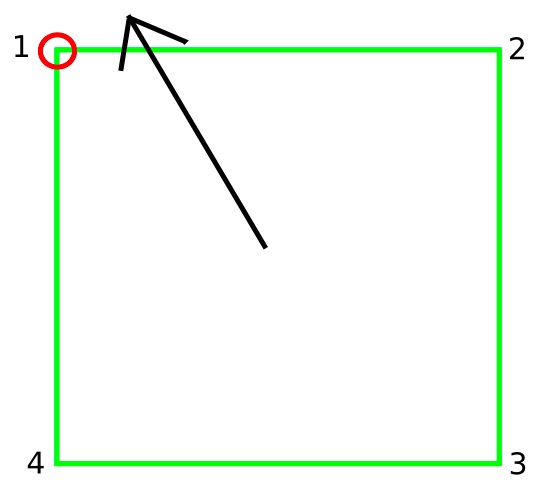In case one, point one is the farthest point on the object along the search direction. If you are wondering why we didn't choose the point on the line where the arrow crosses the square, tilt your head so the arrow is pointing straight up. See? The corner really is the farthest point in that direction.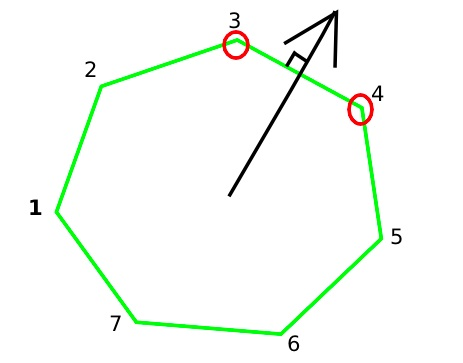In case two, the search direction is normal to the side so both points 3 and 4 are valid. The algorithm will work regardless of which point you pick.

Here's the awesome part. Rather than explicitly compute all the points of the Minkowski object and then calling our support function on that, we can simply call the support function on each of our two simple polygonal objects and compute the appropriate Minkowski point using the relationship below. Here's the pseudocode for my support function:

 Function DoSupport() Point on Minkowski along D = (Farthest point on A in direction D) - (Farthest point on B in direction -D) Return Point on Minkowski

VERY IMPORTANT: Observe that we use "-D" in the support function for object B.

Evolving the Simplex

Ive tried to write this consistent with the Molly Rocket presentation which you should really watch if you are serious about implementing this algorithm. The way it works is: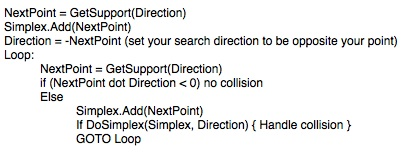That is seriously all there is to it. Well, not quite. What does "DoSimplex" actually do? Remember, we are trying to find the set of simplex points that contains the origin. To do that, we evolve the simplex to always contain those features closest to the origin, and to pick a new direction for us to search for the next candidate point to add to the simplex. Thus our DoSimplex function examines the existing simplex and then sometimes updates the simplex (by removing a point) and always picks the next direction for us to search in. In our formulation we never call DoSimplex on a simplex of size 1, so let's consider the 2-point simplex.

Evolving the Simplex: Two Point Simplex and Voronoi Regions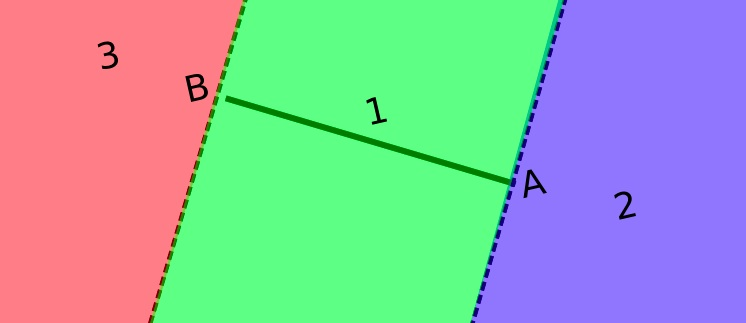In all the pictures, we consider the point A to be the most recently added point. In this case, we picked B and then went searching in our new search direction and found A, the farthest point on the Minkowski difference in that direction. Evolving the simplex means looking for the next search direction and simplifying our simplex. The origin can be in one of the three Voronoi regions defined by this line.

1. The origin can't be in region 3. If it was, there was some other point in region 3 that we would have found instead of point B, because we picked B by saying the farthest point in that direction. Thus we never examine region 3.

2. If we overshot the origin, it is somewhere in region 1. In 2D simply find the perpendicular to the line AB in the direction of the origin because we know the origin still lies in the plane of the line. in 3D, you need to use the triple cross product to get the perpendicular to the line AB in the direction of the origin. The test "Did we overshoot the origin is simply the dot product of vector AB with A0. If the dot product is greater than 0, then the angle between AB and A0 is less than 90 degrees, putting it in on the region 1 side of the plane divided by the dotted line at A, which could be region 1 or 3. As we know it is not region 3, it must be region 2.

3. If we went as far as we could in our search direction and couldn't pass the origin, we know the origin is still somewhere farther than Point A, so it must still in region 2. We can drop B from our simplex because it is not useful and set our new search direction to be from A towards the origin.

Evolving the Simplex: Three Point Simplex and Voronoi Regions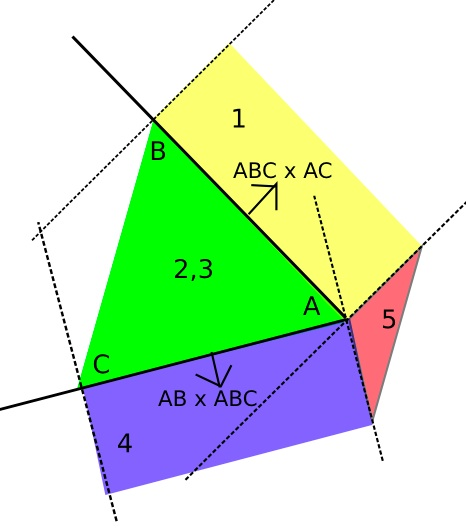This looks a lot more complicated, but it isn't, not really. It is essentially two line simplexes with a bit of reasoning about the other regions. First, observe the white regions to the left of the drawing. We can reject those by the same reasoning we rejected the region behind B in the line simplex case: We are only considering this point A because we already decided it was more in the direction of the origin than anything on the other side of the line BC, so why would we waste work looking regions we already know are farther away from the origin?

We evolve our simplex in the following manner: First we check to see if the origin is on the side of the AB line away from C. If so, we can ignore everything on the C side of the AB line, and then just check to see if the origin is in the yellow part or the pink part of that side of the line. Because C can't be part of an optimal simplex, we remove it from the simplex. In the same way, we can check the area on the side of the AC line away from B, and if it is, decide if the origin is in the blue or the pink side of that area. Because B can no longer be part of an optimal simplex, we remove it instead.

If the origin is not in either the yellow, blue, or pink regions, then it must be in the central green region. If it is, we have surrounded the origin, thus detecting a collision.

Actually, we are only done in 2D. In the 3D case the origin may still lie above the plane or below the plane. The MollyRocket video covers it but I do not. It is an obvious continuation of the simplex evolution strategy, but now using a tetrahedron (a 3D simplex).

One important thing to note is that the MollyRocket video and these notes use ABC to denote the triangle normal. The cross product checks that use the triangle normal need to be the vector that points to the outside of the triangle (shown in the image above). The triangle normal ABC is computed as AB x AC. Draw a couple triangles to convince yourself no matter how you assign the points A,B and C, you will compute vectors pointing "out" in this fashion.

EPA: What to do after penetration is detected

The second part of a physics engine, after collision detection, is collision resolution, where we use the information about the collision to correct the velocities of the objects. This is a considerable subject in its own right, but for now, I will limit the discussion to only the problem of How do you use GJK when you want to know the contact point and collision depth? When we detect a penetration (the case where we return true in the pseudocode, region (2,3) in our triangle simplex), then we must use another algorithm to find that information. One good one is Expanding Polytope Algorithm (EPA). Another is Separating Axis Test (SAT). SAT can actually replace GJK entirely, but it is not quite as fast and doesnt scale well to 3D objects so it is less commonly used. I will cover neither of these here but will provide a link to an example of 2D EPA.

Code listing

I have included a code listing. It won't compile to anything meaningful out of the box because it uses the Havok SDK but it should be fairly readable and demonstrate the concepts shown here.

References

I've discovered a lot of great resources online while researching my own implementation of GJK. The best explanation of GJK is at the MollyRocket link, but the others also contain useful information. There is a mostly correct GJK explanation at codezealot, but the author takes a couple of shortcuts like not checking his triangle normals that will trip the unwary observer. His simplex.getA(), .getB() and .getC() methods return the appropriate vertex to preserve the orientation of the triangle normal which is an inappropriate simplification in a pedagogical exercise such as this.